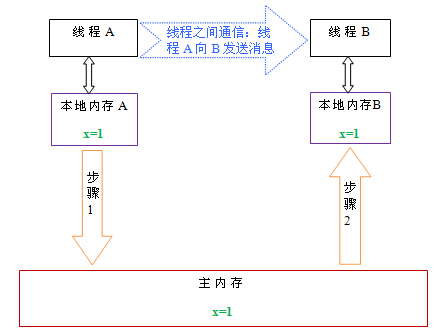## 问题的背景

### CPU 缓存## 内存模型解决了什么问题

r2=A; //1 r1=B; //3
B=1; //2 A=2; //4

1. 执行顺序假设是：1->2->3->4，答案是 r1=1 r2=0
2. 执行顺序假设是：1->3->2->4，1->3->4->2，3->1->4->2，3->1->2->4，答案是 r1=0 r2=0
3. 执行顺序假设是：3->4->1->2，答案是 r1=0 r2=2